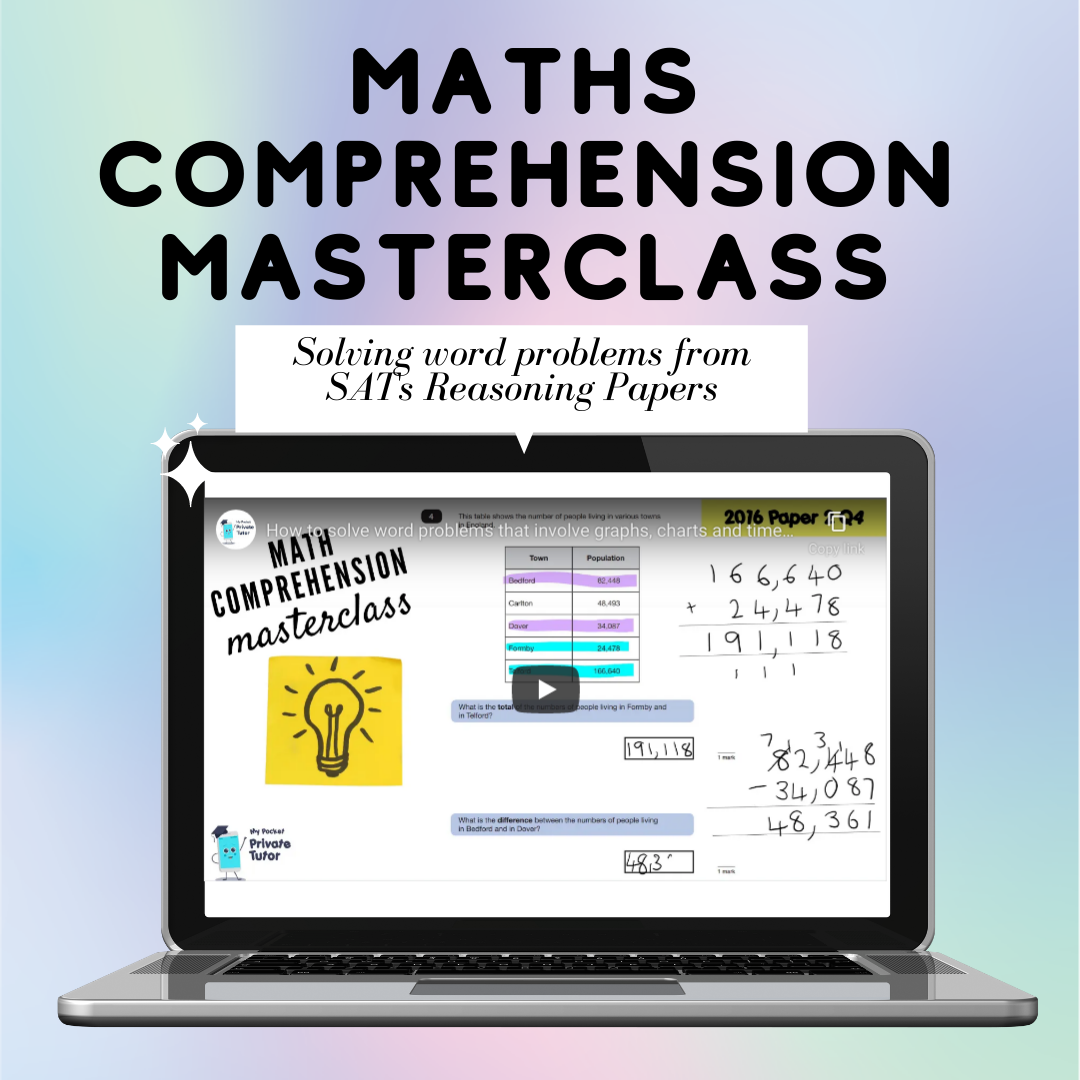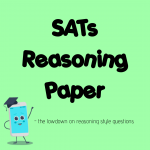## Why is a word problem hard in maths?

A math word problem is when a problem is presented using a real life scenario and a child has to work out what maths they need to do to find the solution. It is so common to hear that a child knows how to add, subtract, multiply and divide confidently but then when they get to a word problem it all goes out of the window! Some of it is a mental block, they might not understand the problem, there could be a lack of familiarity with the format of questions, not enough taught strategies for solving word problems or all of the above! Word problems are found in SATs Papers 2 & 3 and are generally the harder papers to pick points up in.

## What is the difference between arithmetic questions and reasoning questions?

Take a look at these comparisons of the same math skill in the formats of each type of paper.

In both of these examples a percentage of an amount needs to be calculated, it is obvious in  Q27 but in Q18, children need to read the scenario and work out that they need to first find the percentage but then subtract this answer from the £15 t-shirt. It would not be uncommon for children to find the 30% of £15 and leave that as their final answer here.

Both of the following questions from SATs 2022 papers involve a long division calculation. A common misconception here would be for children to multiply 850ml by 17 instead of divide.

The next two questions involve multiplying a mixed number by a whole number. In each question, the same strategy of partitioning the whole number and the fraction and then multiplying by the whole number could be used. For the arithmetic question, children could also convert the fraction to a decimal and use their understanding of multiplying by 10. For the reasoning problem, children might solve by adding the full priced box to a half price box. For a question like this I would always recommend annotating the question. I would get them to label the price of the 2nd box. Then it is far easier to see that you just need to add the two prices together.

The following two questions are similar in that they have multiple steps. Q15 clearly sets out the order using brackets. there are no brackets in Q3 but using them would be helpful. For Q3 I would encourage children to write out the number sentence as (3 x 50) – (30+45+60)=

The final 2 examples require a number to be multiplied by 1000. In Q29, it is obvious that’s what you need to do, it is less obvious in Q5 unless a child uses a strategy to represent and understand what is being asked.

## Why does my child find a word problem harder in a test?

In class, children are told what they are learning. They start the lesson with a lesson objective, for example adding fractions. When they are then presented with a word problem they already have a clue about what math skill to apply. In a test however, they are given a variety of word problems covering a variety of math skills. They need to make decisions based on the words in the problem about what math skills they need to use. This is where children can have mental blocks or act hastily. Often they will use one step correctly but then not follow through with the next step or perhaps carry out the write calculation but use the wrong part of their workings for the answer. It is really common!

## How do I help my child get confident with solving a word problem?

The best way to improve confidence is lots of exposure! Practice really does make perfect. Children will need to learn about the vocabulary used and take their time. For example, when they see the word less they often jump to the conclusion that they need to subtract but if the problem is,

Mr A has saved £50. He had £10 less than Mr B. How much does Mr B have?

the problem actually requires adding not subtracting. Bar models are a great tool for representing word problems in a way that helps a child to see what calculating they need to do. White Rose have a great bank of free resources using problems with bar models for every year group. Use these for practice. Or you can purchase one of my Bar Model Courses, there is one available for Years 3&4 and another for years 5&6. Each course runs through HOW to use bar models to help solve word problems.

Children should be taught a range of  strategies such as drawing diagrams, using number lines, underlining important words and numbers but most importantly, they need feedback. There’s no point in them receiving problems back that are marked incorrectly, the learning comes from having it explained and demonstrated. I have a free video on my YouTube channel that runs through some common strategies for word problems. Click here to view

You can also purchase my Maths Comprehension Masterclass for just £9.99This is a 5 part course with video tutorials and printable worksheets. Learn how to solve common word problems found in the Year 6 SATs Reasoning Papers. The course is a digital download which you can view on your laptop, tablet or phone. You can even cast the video tutorials onto a smart TV. Each of the 5 parts explores a different category of reasoning problem and demonstrates ways to approach solving the problem. Real past SATs questions are used to demonstrate.

## Use maths in real life

A way to develop your child’s maths problem solving skills is to build it into your everyday life. Discuss time durations….how long until? How long did it take? What time will it be in 90 minutes etc… When you go shopping encourage your children to  figure out the cheaper option. Get them involved with calculating change or how to split a bill in a restaurant. The problem is we do so much with our cards these days that children are not exposed to receiving change as much these days but it doesn’t mean you can’t still discuss the question. When cooking, get them to think about how to change a recipe quantity for different servings. Get kids involved with budgeting….next time you pop to McDonalds- tell them they have a certain amount to spend and see what they can buy for that. The more they get used to solving everyday real life math problems, the less abstract the word problems will be. Plus it’s building real life skills anyway!For some more info on reasoning style questions you can also take a look at my blog